# Database Normalization

This article explains about database normalization which is helpful when discussing the design of a relational database. This article also describes what is normalization and why it is necessary. It also demonstrates about the types of normalization and its pros and cons. Let’s start with simple definition of normalization.

What is Anamoly?

Now this question must have come in your mind that what is anomaly? Anomalies are problems that can occur in poorly planned, un-normalised databases where all the data is stored in one table (a flat-file database). There are three types of anomalies that occur when the database is not normalized. These are — Insertion, update and deletion anomaly. Let’s take an example to understand this.

Suppose a college stores the student details in a table named student that has four attributes: student_id for storing student’s id, student_name for storing student’s name, student_address for storing student’s address and student_dept for storing the department details it which the student is associated. At some point of time the table looks like this:

The above table is not normalized. We will see the problems that we face when a table is not normalized.

Update anomaly: In the above table we have two rows for student Rohit as he belongs to two departments of the company. If we want to update the address of Rohit then we have to update the same in two rows or the data will become inconsistent. If somehow, the correct address gets updated in one department but not in other then as per the database, Rohit would be having two different addresses, which is not correct and would lead to inconsistent data.

Insert anomaly: Suppose a new student joins the college but he is not assigned to any department yet. Then we would not be able to insert the data into the table if student_dept field doesn’t allow nulls.

Delete anomaly: Suppose, if at a point of time the company closes the department CSE then deleting the rows that are having student_dept as CSE would also delete the information of student Sachin since he is assigned only to this department.

To overcome these anomalies we need to normalize the data. In the next section we will discuss about normalization.

What is Normalization?

Normalization is a process of organizing the data in database to avoid data redundancy. Redundancy in relation may cause insertion anomaly, update anomaly & deletion anomaly. Or We can also say that Normalization in DBMS is a technique that helps in designing the schema of the database to ensure that the duplication is minimized. Normal forms are used to eliminate or reduce redundancy in database tables. Here are the most commonly used normal forms:

1. First normal form(1NF) :- First normal form (1NF) is a property of a relation in a relational database. A relation is in first normal form if and only if column contains only single value. It should hold only atomic values.

Example: Suppose a college wants to store the names and contact details of its students. It creates a table that looks like this: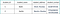Student Table

One Student Sachin is having two mobile numbers so the college stored them in the same field as you can see in the table above.

This table is not in 1NF as the rule says “each column of a table must have atomic (single) values”, the student_contact values for student Sachin violates that rule.

To make the table complies with 1NF we should have the data like this: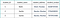Student Table

2. Second normal form (2NF) :- Second normal form (2NF) is a property of a relation in a relational database. A relation is in second normal form if and only if table hold both the following conditions:

— Table is in 1NF (First normal form)
— No non-prime attribute is dependent on the proper subset of any candidate key of table.

An attribute that is not part of any candidate key is known as non-prime attribute.

Example: Suppose a college wants to store the data of teachers and the subjects they teach. They create a table that looks like this: Since a teacher can teach more than one subjects, the table can have multiple rows for a same teacher. Here teacher_id,teacher_age are candidate keys and teacher_age is non prime attribute.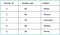Teacher Details Table

The table is in 1 NF because each column has atomic values. However, it is not in 2NF because non prime column teacher_age is dependent on teacher_id alone which is a proper subset of candidate key. This violates the rule for 2NF as the rule says “no non-prime attribute is dependent on the proper subset of any candidate key of the table”.

To make the table complies with 2NF we can break it in two tables i.e. teacher_details & teacher_subject tables like: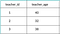Teacher Details Table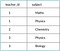Teacher Subject Table

Now the tables comply with Second normal form (2NF).

3. Third Normal form (3NF) :- A table design is said to be in 3NF if both the following conditions hold:

— Table must be in 2NF.
— Transitive functional dependency of non-prime attribute on any super key should be removed.

An attribute that is not part of any candidate key is known as non-prime attribute.

Example: Suppose a college wants to store the complete address of each student, they create a table named student_details where student_id is candidate key. All columns except student_id are non-prime as they are not part of any candidate keys.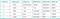Student Details Table

Here, student_state, student_city & student_district dependent on student_zip. And, student_zip is dependent on student_id that makes non-prime attributes (student_state, student_city & student_district) transitively dependent on super key (student_id). This violates the rule of 3NF.

To make this table complies with 3NF we have to break the table into two tables i.e. student and student_zip to remove the transitive dependency: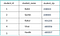Student table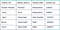Student Zip Table

4. Boyce Codd normal form (BCNF) :- It is an enhance version of 3NF that’s why it is also known as 3.5NF. BCNF is stricter than 3NF. A table complies with BCNF if it is in 3NF and for every functional dependency X->Y, X should be the super key of the table.

Example: Suppose there is a college where in students study in more than one department. They store the data like this where student_id & student_dept are candidate keys: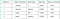Student Table

Functional dependencies in the table above:
student_id -> student_nationality
student_dept -> {dept_type, dept_no_of_student}

The table is not in BCNF as neither student_id nor student_dept alone are keys.

To make the table comply with BCNF we can break the table in three tables i.e. student_nationality, student_dept & student_dept_mapping: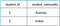Student Nationality Table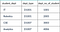Student Dept Table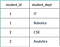Student Dept Mapping Table

Functional dependencies:
student_id -> student_nationality
student_dept -> {dept_type, dept_no_of_emp}

Candidate keys:
For first table: student_id
For second table: student_dept
For third table: {student_id, student_dept}

This is now in BCNF as in both the functional dependencies left side part is a key.

With this, we come to an end to this blog. I hope you got a clear understanding about Normalization and its types from this article.